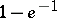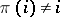# Montmort matching problem

(diff) ← Older revision | Latest revision (diff) | Newer revision → (diff)
Jump to: navigation, search

derangement problem

Take two sets,and, and a bijection,, between them. (E.g., takemarried couples and letbe the set of husbands andthe set of wives.) Now, take a random paring (a bijection again). What is the chance that this random pairing gives at least one "correct match" (i.e. coincides within at least one element). Asymptotically, this probability is. This follows immediately from the formula given in Classical combinatorial problems for the number of permutationssuch thatfor all.

This problem was considered first by P.R. de Montmort (around 1700) in connection with a card game known as the "jeu du treize" , "jeu de rencontre" or simply "rencontre" .

How to Cite This Entry:
Montmort matching problem. Encyclopedia of Mathematics. URL: http://encyclopediaofmath.org/index.php?title=Montmort_matching_problem&oldid=14268
This article was adapted from an original article by M. Hazewinkel (originator), which appeared in Encyclopedia of Mathematics - ISBN 1402006098. See original article